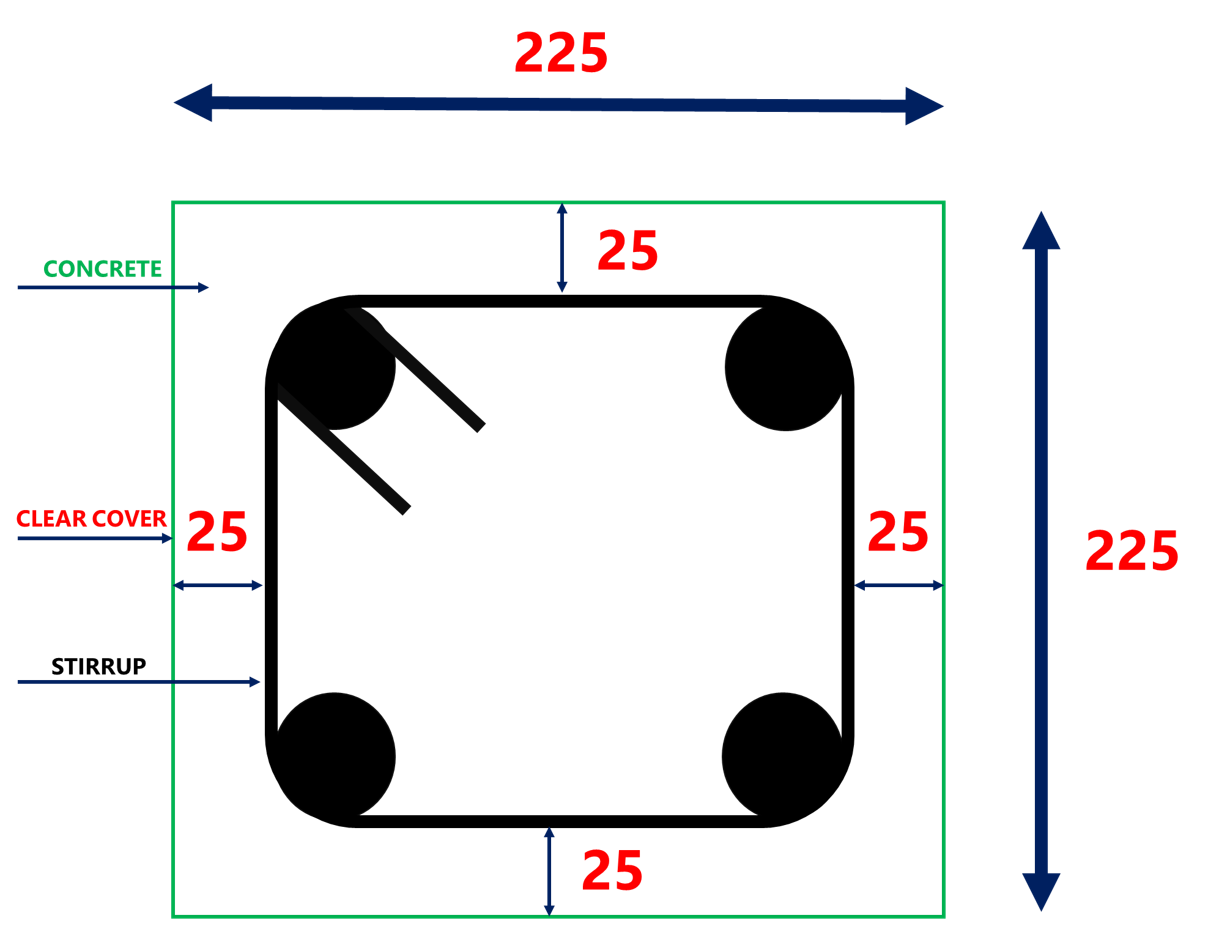HOW TO CALCULATE CUTTING LENGTH FOR SQUARE STIRRUPS -lceted LCETED INSTITUTE FOR CIVIL ENGINEERS

## HOW TO CALCULATE CUTTING LENGTH OF SQUARE STIRRUPS IN BEAM AND COLUMNHOW TO CALCULATE CUTTING LENGTH OF STIRRUPS ?

A stirrup is a closed rebar loop that is used to hold the main rebar together in an RCC structure. In one column, the stirrups provide lateral support to the main bars against buckling.

Different Shapes of Stirrups

1)     Rectangular Stirrups

2)     Square Stirrups

6)     Diamond Stirrups

Types Of Stirrups Used In Beam And Columns

1)     Single Legged Stirrups(Open stirrup)
2)     Two-legged or Double Legged Stirrups (Closed Stirrup)
3)     Four-legged Stirrups (Closed Stirrup)
4)     6-legged Stirrups (Closed Stirrup)

Steps involved in finding the cutting length of stirrups

1)     Look at the size of column or beam from drawings
2)     Adopt Dia of the bar (generally 8mm Dia is used for stirrups)
3)     Deduct the concrete cover or clear cover
4)     Find the total outer length of stirrup after deducting concrete cover.
5)     Add the length of the hook to the length of the stirrup
6)     Deduct the length of bends
7)     Use below formula to find the total cutting length of stirrups

Formula To Find The Total Cutting Length Of Stirrups
Cutting Length of Stirrups = Perimeter of Shape + Total hook length – Total Bend Length

Perimeter Of Shape
Perimeter of Rectangle = 2 ( length + breadth)
Perimeter of Square = 4 x side length
Perimeter of circle or Circumference of Circle = 2πr = πd
(r= radius, d= Diameter of Circle)
Total hook Length
1 Hook length = 9d or 75mm
Total Bend Length
45° Bend length = 1d
90° Bend length = 2d
135° Bend length = 3d (Remember, d = Diameter of Bar)

## Cutting Length of Square Stirrups

Cutting Length of Stirrups = Perimeter of Shape + Total hook length – Total Bend Length

Cutting Length of Square Stirrup = 4a + 2 numbers of hooks – 3 numbers of 90° bends – 2 numbers of 135° bends

EXAMPLE SQUARE STIRRUP CALCULATION

1.   We can Consider the column size as 225mm x 225mm

2.   Adopting Dia of Bar used for stirrups is 8mm (d=8mm)

3.   Deducting the concrete cover 25mm from all sides (in the square all sides are equal)
a = 225- 25-25 = 175mm
b = 225-25-25 = 175mm, Hence a = b (in square all sides are equal)

4.   Total Length of the hook:
There are 2 hooks which mean 9d+9d = 18d
5.   Total length of Bends:
There are 3 bends which are bent at an angle of 900 and 2 bends at an angle of 1350.

Total Cutting Length of Square Stirrup

= 4a + 2 numbers of hooks – 3 numbers of 90° bends – 2 numbers of 135° bends

= 4 x 175 + (18d) – (3 x 2d) – (2 x 3d)
(d=8mm as we mention step -2)
= 700 + (18 x 8) – (3 x 2×8) – (2 x 3 x 8)
= 700+ (144) – (48) – (48)

=748mm = 0.748m

Total Cutting Length of Square Stirrup = 0.748m

LCETED INSTITUTE FOR CIVIL ENGINEERS

Our Mission To Educate And Keep People Informed By Creating A Trusted Source Of Knowledge Everything Related To Civil Engineering”.

LCETED SUGGESTS YOU READ THIS TOPIC ALSO:

## LCETED - "WHATSAPP GROUP"

For Instant updates Join our Whatsapp Group. Save our Whatsapp contact +919840140396 as LCETED and Send us a message “JOIN”